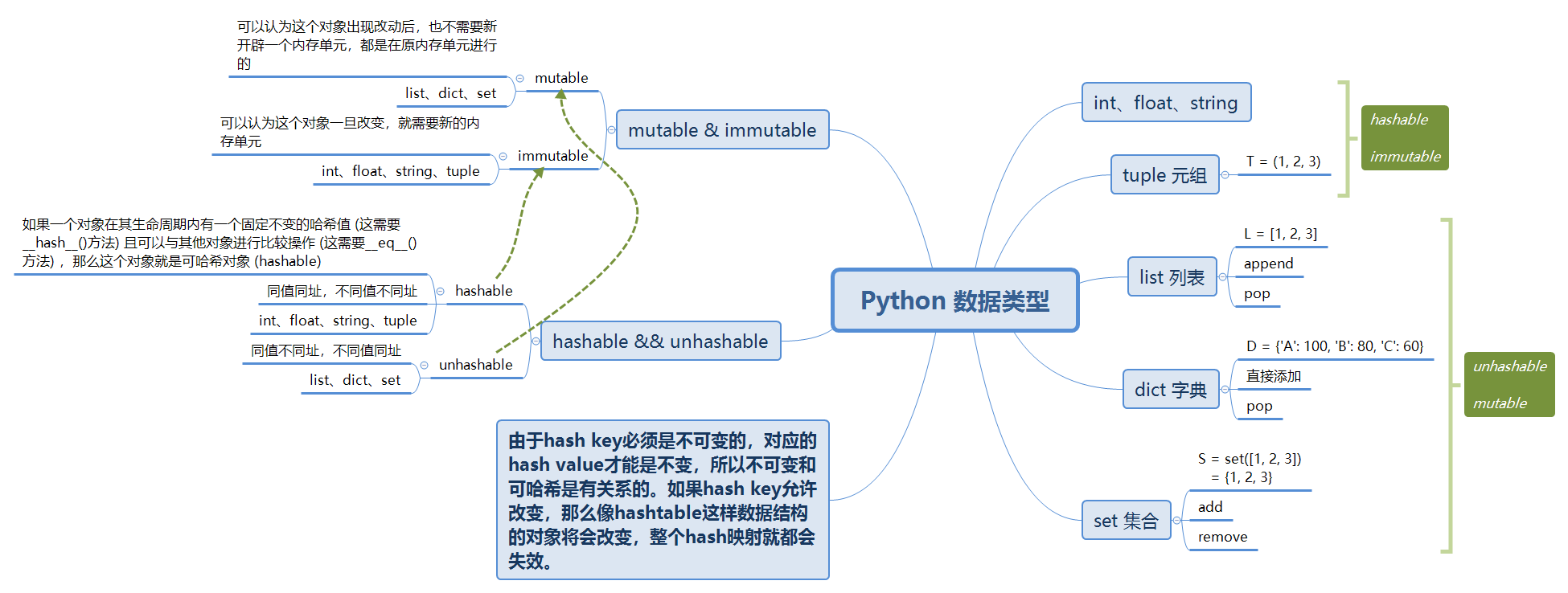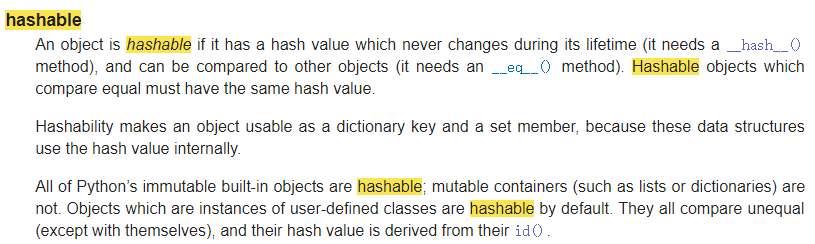# Python学习笔记 | 关于python数据对象 hashable & unhashable 的理解

### 文章目录### 写在前面

Hash（哈希、散列）是一个将大体量数据转化为很小数据的过程，甚至可以仅仅是一个数字，以便我们可以在O(1)的时间复杂度下查询它，所以，哈希对高效的算法和数据结构很重要。

immutable（不可改变性）是指一些对象在被创建之后不会因为某些方式改变，特别是针对任何可以改变哈希对象的哈希值的方式。

### hashable & unhashable### 实例检测

#!/usr/bin/env python
# -*- coding: utf-8 -*-

# unhashable 可变对象
# 如list、dict、set：同值不同址，不同值同址

# hashable 不可变对象
# 如int、str、char、tuple：同值同址，不同值不同址

# 怎么判断可变不可变 ？
# 改个值，看id是不是一样，id一样的为可变，则不可哈希。id出现变化，则为不可变，可哈希

# list
L = [1, 2, 3]
L2 = [1, 2, 3]
print('id(L)', id(L))
print('id(L2)', id(L2))
L = 4
print('id(L)', id(L))   # unhashable

'''---------------------
id(L) 2763485176456
id(L2) 2763485176520
id(L) 2763485176456
---------------------'''

# dict
D = {'A':100, 'A-':90, 'B':80, 'C':70}
D2 = {'A':100, 'A-':90, 'B':80, 'C':70}
print('id(D)', id(D))
print('id(D2)', id(D2))
D['A'] = 99
print('id(D)', id(D))   # unhashable

'''---------------------
id(D) 2763485641608
id(D2) 2763485641680
id(D) 2763485641608
---------------------'''

# set
S = set([1, 2, 3])
S2 = set([1, 2, 3])
print('id(S)', id(S))
print('id(S2)', id(S2))
S.remove(1)
print('id(S)', id(S))   # unhashable

'''---------------------
id(S) 1905131096776
id(S2) 1905131094088
id(S) 1905131096776
---------------------'''

# int
a = 666
b = 666
print('hash(a):', hash(a))
print('hash(b):', hash(b))
print('id(a)', id(a))
print('id(b)', id(b))
a = 555
print('hash(a):', hash(a))
print('id(a)', id(a))   # hashable

'''---------------------
hash(a): 666
hash(b): 666
id(a) 1905130526128
id(b) 1905130526128
hash(a): 555
id(a) 1905131082192
---------------------'''

# float
a = 1.2
b = 1.2
print('hash(a):', hash(a))
print('hash(b):', hash(b))
print('id(a)', id(a))
print('id(b)', id(b))
a = 1.1
print('hash(a):', hash(a))
print('id(a)', id(a))   # hashable

'''---------------------
hash(a): 461168601842738689
hash(b): 461168601842738689
id(a) 2682206597600
id(b) 2682206597600
hash(a): 230584300921369601
id(a) 2682206597624
---------------------'''

# str
c = 'ZJ'
d = 'ZJ'
print('id(c)', id(c))
print('id(d)', id(d))
c = 'YF'
print('id(a)', id(c))   # hashable

'''---------------------
hash(c): 3106900240887856397
hash(d): 3106900240887856397
id(c) 1642181677384
id(d) 1642181677384
hash(c): -2749512413466868010
id(c) 1642181677608
---------------------'''

# tuple
T = (1, 2, 3)
T2 = (1, 2, 3)
print('hash(T):', hash(T))
print('hash(T2):', hash(T2))
print('id(T)', id(T))
print('id(T2)', id(T2))

'''---------------------
hash(T): 2528502973977326415
hash(T2): 2528502973977326415
id(T) 2325794115656
id(T2) 2325794115656
---------------------'''

# tuple2
T = (1, [1, 2], 3)
T2 = (1, [1, 2], 3)
print('id(T)', id(T))
print('id(T2)', id(T2))
T = 4
print('id(T)', id(T))   # hashable

'''---------------------
id(T) 2979746926664
id(T2) 2979747407048
id(T) 2979746926664
---------------------'''

# 补充说明
# 虽然字符串有个replace()方法,也确实变出了'Abc',但变量a最后仍是'abc'
a = 'abc'
print('a:', a)
print('a:', a.replace('a', 'A'))
print('a:', a)

'''---------------------
a: abc
a: Abc
a: abc
---------------------'''

# 这是因为 a.replace('a', 'A') 相当于
b = a.replace('a', 'A')     # replace方法创建了一个新字符串'Abc'并返回

# Summary:
# 对于不变对象来说, 调用对象自身的任意方法, 也不会改变该对象自身的内容。
# 相反, 这些方法会创建新的对象并返回, 这样, 就保证了不可变对象本身永远是不可变的。

>>> class A:
...     pass
...
>>> cls_a = A()
>>> cls_b = A()
>>> cls_a
<A object at 0x000001890DB69898>
>>> cls_b
<A object at 0x000001890DB6EDD8>
>>> cls_a.__hash__()
-9223371931345262199
>>> cls_b.__hash__()
-9223371931345260835
>>> id(cls_a)
1688152217752
>>> id(cls_b)
1688152239576
# 这里两个对象（cls_a和cls_b）哈希值和id都不一样
# 由于用户自定义的类的实例其哈希值与id有关，所以id值和哈希值都不相同，就如官方文档里说的，实例只跟自己相等。


Python内置的可哈希对象可以使用hash()或者__hash__()方法来查看它的哈希值，如：a.__hash__()或者hash(a)，而id()函数用于获取对象的内存地址。

### 后续思考

tuple虽然是不可变对象，但试试把(1, 2, 3)(1, [2, 3])放入dictset

# tuple是不变对象，试试把(1, 2, 3)和(1, [2, 3])放入dict或set中

T = (1, 2, 3)
T2 = (1, [2, 3])

D = {T: 100}
print('D:', D)

'''-----------------
D: {(1, 2, 3): 100}
-----------------'''

# D2 = {T2: 100}      # TypeError: unhashable type: 'list'
# print('D2:', D2)

S = set([T])
print('S:', S)

'''-----------------
S: {(1, 2, 3)}
-----------------'''

# S = set([T2])       # TypeError: unhashable type: 'list'
# print('S:', S)


### 参考文章posted @ 2019-04-01 22:32  zhouie  阅读(1651)  评论(0编辑  收藏  举报